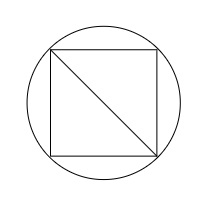# A square of diagonal 8 cm is inscribed in a circle. Find the area of the region lying inside the circle and outside the square.

Given:

A square of diagonal 8 cm is inscribed in a circle.

To do:

We have to find the area of the region lying inside the circle and outside the square.

Solution:Let the side of the square be $a$ and the radius of the circle be $r$.

The length of the diagonal of the square $= 8\ cm$

Diagonal of the square of side $a=\sqrt{2} a$

This implies

$\sqrt{2}a=8$

$\Rightarrow a=4 \sqrt{2} \mathrm{~cm}$

Diagonal of the square $=$ Diameter of the circle

This implies,

Diameter of the circle $=8\ cm$

Radius of the circle $r=\frac{\text { Diameter }}{2}$

$\Rightarrow r=\frac{8}{2}$

$\Rightarrow r=4 \mathrm{~cm}$

Therefore,

Area of the circle $=\pi r^{2}$

$=\pi(4)^{2}$

$=16 \pi \mathrm{cm}^{2}$

Area of the square $=a^{2}$

$=(4 \sqrt{2})^{2}$

$=32 \mathrm{~cm}^{2}$

The area of the region lying inside the circle and outside the square $=$ Area of the circle $-$ Area of the square

$=(16 \pi-32) \mathrm{cm}^{2}$
The area of the region lying inside the circle and outside the square is $(16 \pi-32) \mathrm{cm}^{2}$.